Conducting the Dose–Response Assessment

Chapter 8
Conducting the Dose–Response Assessment

The objective of dose–response assessment is to develop a relationship between the level of microbial exposure and the likelihood of occurrence of an adverse consequence. In general, dose–response analysis would not be necessary if the level of microbial risk that was acceptable was sufficiently high so that actual experimentation (on humans or perhaps on animals) could be conducted so as to permit the direct assessment of risk in the observable range. However, since frequently the level of risk from a single exposure is less than 1/1000, the use of direct experimentation to assess this risk becomes impractical (as well as ethically questionable) since >1000 subjects would be needed to ascertain the “acceptable” dose. Therefore, the use of a parametric dose–response curve to facilitate low-dose (and low-risk) extrapolation becomes necessary.

A dose–response model is, in a most general sense, a mathematical function that takes as an argument a measure of dose, which can be any non-negative number, and yields the probability of the particular adverse effect, which is bounded by zero (no effect) and one (complete conversion to adverse state). There are an infinite number of such possible functions. Even restricting the universe to those functions that are monotonic (as dose increases, response probability is nondecreasing) and bounded by zero and one (at dose = 0, no response; as dose = 1, complete response), there remain an infinite number of possible functions. In particular, the last set of conditions is identical to those required of cdfs, so any cdf with support over the range 〈0,〉 can be a candidate dose–response function. Conversely, any dose–response function that is monotonic and bounded by zero and one with support over the range 〈0,〉 is a cdf—this is convenient, since for many distribution functions, the mathematical properties have been well studied.

It is most desirable that the dose–response model(s) chosen for use is biologically plausible. Therefore, we will first review some attributes of a biologically plausible microbial dose–response model for infectious agents. Then some classes of these models will be derived and analyzed. Subsequently, some empirical (albeit lacking in plausibility) models will be presented. The remainder of this chapter will then deal with issues of fitting data to models and assessing validity using independent data.

Plausible Dose–Response Models

There are two key distinguishing features of infectious microorganisms from other human health risks (e.g., chemicals, ionizing radiation). Any dose–response model failing to consider these factors lacks biological plausibility.

First, particularly at low levels, the statistics of microbial distribution (as discussed in Chapter 7) dictate that a population of humans exposed to infectious agents will of necessity receive a distribution of actual doses. For example, if a group of people each consume exactly 1 l of water containing an average concentration of 0.1 organisms per liter, we expect (assuming random Poisson distributions between doses) that about 90% of persons (exp(−0.1)) would actually consume zero organisms, about 9% of persons (0.1 × exp(−0.1)) would consume one organism, 0.45% of persons would consume two organisms, and 0.015% would consume three or more organisms. If the distribution of organisms between doses was nonrandom (e.g., NB), then the percentages would obviously be different. Any biologically plausible dose–response framework should consider this phenomenon. This is very different than with many chemicals, which are present at high enough concentrations (when viewed in terms of molecules) such that the quantal nature of the delivered substance does not affect the actual delivered dose between subjects exposed.

The second distinguishing aspect of infectious microbial agents is their ability to propagate within a susceptible host at an appropriate location within the body. There are, in fact, suggestions that the very trait of microbial pathogenicity may result from coevolutionary processes between infectious agents and humans . Although there are quite specific mechanisms of pathogenicity and methods of circumventing the numerous antimicrobial defense mechanisms of the human body, the infection and disease process represent an overcoming of these barriers by the infectious agent . The time course of microbial infection can be described by competing processes of birth and death within the host, infection resulting when birth is sufficient to produce a body burden above some critical level to induce the effect .

The exposure to microbial agents can result in a series of endpoints:

• Infection
• Illness
• Severe illness
• Death

A fraction of individuals, PI, will become infected. Infection is manifest as the multiplication of organisms within the host, followed by excretion. In addition, measurable rises in serum antibodies or increase in body temperature can indicate infection. Of those infected, a fraction of these, PD:I (termed the morbidity ratio), will become ill. Of those becoming ill, a fraction of these, PM:D (termed the mortality ratio), will die.

For some infectious agents, the development of consequences can be quite complex and involve multiple disease symptomatologies and endpoints. This is illustrated in Figure 8.1 for the case of Escherichia coli O157:H7, based on overall U.S. prevalence statistics . This figure illustrates another aspect of many infectious agents; often, the frequency of more severe consequences is better characterized than the frequency of less severe endpoints, including infection, since the latter endpoints represent individuals who may not be recognized by the health care system (and they may not seek medical attention).

In dose–response assessment, we will focus heavily on the early processes, particularly infection. The relationship of PI to dose will be characterized. Focus on infection as an initial object of study is justified since, from a public health point of view, the minimization of infections may introduce an element of conservatism into the analysis.

Framework for Mechanistic Dose–Response Relationships

The process of infection may be considered as requiring two sequential subprocesses to occur :

1. The human host must ingest one or more organisms that are capable of causing disease.
2. The organisms undergo decay or are impaired from multiplying to cause infection/disease by host responses, and only a fraction of the ingested organisms reach a site where infection can commence.

The probability of ingesting precisely j organisms from an exposure in which the mean dose (perhaps the product of volume and density, both estimated via the methods previously described) is d (i.e., the first of the sequential processes) is written as P1( j|d). The probability of k organisms (≤j) surviving to initiate an infectious process (the second step) is written as P2 (k|j). If these two processes are regarded as independent, then the overall probability of k organisms surviving to initiate infectious foci is given by (the law of independent events)

(8.1)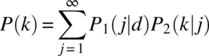The function P1 incorporates the individual-to-individual variation in actual numbers of organisms ingested or otherwise exposed, while the function P2 expresses the factors of organism–host interaction in vivo that allow some organisms to survive to initiate infectious foci.

Infection occurs when at least a critical number of organisms survive to initiate infection. If this minimum number is denoted as kmin, then the probability of infection (i.e., the fraction of subjects who are exposed to an average dose d who become infected) may be written as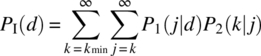We emphasize that the use of kmin in the precise sense of Equation (8.2) does not correspond to the often used term “minimal infectious dose” . The latter term refers to the average dose administered and most frequently really relates to the average dose required to cause half of the subjects to experience a response; we would prefer the term median infectious dose for this. As we will show, one cannot ipso facto infer from the magnitude of the median infectious dose whether or not kmin is large or small (as small as 1). The following observation of Meynell and Stocker  is illuminating:

The two conceptual alternatives have been termed the hypothesis of independent action, in which in principle kmin equals 1, and the hypothesis of cooperative interaction, in which kmin is some number greater than 1.

If it is understood that kmin may not be a single number but may in fact be a probability distribution (we will generalize Equation (8.2) to admit this possibility subsequently), then Equation (8.2) or a generalization of 8.2 is expected to be sufficiently broad to encompass all plausible dose–response models. By specifying functional forms for P1 and P2, as well as numerical values of kmin, we can derive a number of specific useful dose–response relationships.

Exponential Dose–Response Model

The simplest dose–response model that can be formulated assumes that the distribution of organisms between doses is random, that is, Poisson, that each organism has an independent and identical survival probability,1 r, and that kmin equals 1. From the Poisson assumption, we haveThe assumption with respect to survival means that the binomial distribution can be used as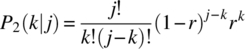Substituting Equations 8.3 and 8.4 into Equation (8.2) yields

(8.5)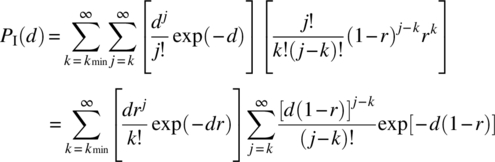The second summation equals unity (it is the sum of a Poisson series), and therefore, we haveFinally, with the assumption of kmin = 1, this yields2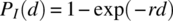This is the exponential dose–response relationship. It has one parameter, r, which characterizes the process. The median infectious dose (N50) can be given by

(8.8)Note that the mean dose is multiplied by a constant. This in effect means that any measurement of microbial dose can be used, which is strictly proportional to microbial dose. Given the fact that, as noted in Chapter 5, some measurement methods may be imperfect, this means that we can nonetheless use these methods for dose–response assessment with the exponential model, recognizing that the parameter, r, is specific for that method. This same observation will hold true for the other dose–response models to be considered in the following text.

The exponential dose–response relationship has the property of low-dose linearity. If rd « 1, then exp(−rd) ≈ 1 − rd, and Equation (8.7) can be approximated as

(8.9)Another property of this and other dose–response curves that we will examine is the slope of the curve at the median point (PI = 0.5). Differentiation of Equation (8.7) produces

(8.10)Since, at the median point, exp(−rd) = 0.5 (see Eq. (8.7)), this can also be written as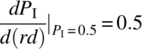By similar analysis, the slope of a log–log plot at the median point for the exponential dose–response equation can be determined to be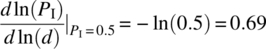Beta-Poisson Dose–Response Model

The exponential model assumes constancy of the pathogen–host survival probability (r). For some agents, and populations of human hosts, there may be variation in this success rate. Such variation may be due to diversity in human responses, diversity of pathogen competence, or both. This variation can be captured by allowing (r) to be governed by a probability distribution. This phenomenon of host variability was perhaps first invoked by Moran . Armitage  appears to have been the first to characterize this variability by a beta distribution; however, computational limitations precluded his use of this model—beta-Poisson and other tolerance distributions. Furomoto and Mickey  appear to be the first to have used this model in the context of microbial dose–response relationships.

To introduce variability in the interaction probability, Equation (8.4) is replaced by a mixture distribution in a similar fashion to the mixture distributions introduced in Chapter 7. However, here (Eq. (8.13)), a mixture distribution of the binomial, with respect to the parameter r, is used:The mixing distribution, f(r), should have (its only) support over the interval <0,1>, corresponding to the allowable range of variability of r itself. Use of Equation (8.13) is identical to applying the mixture operation directly to Equation (8.7), if the Poisson distribution for dose-to-dose variation is assumed, thus yieldingA logical distribution, which offers a great deal of flexibility, is the beta distribution, which for a variable, x, defined between 0 and 1, has a density function ofIncorporating Equation (8.15) into Equation (8.14) yieldsThe integral in Equation (8.16) can be expressed as a confluent hypergeometric function as follows:Properties of this function are given in standard references [13, 14]. In particular, it can be written as a series expansion.

Furomoto and Mickey [11, 12] derived the following approximation to Equation (8.17):The approximation becomes poorer at small values of β or large values of d (and risk). However, both forms (exact and approximate) have the property of low-dose linearity. Furthermore, numerical studies show that the magnitude of discrepancy between the exact and approximate solutions in fitting dose–response data is not substantial  except for some data sets when determining confidence limits. Hence, we shall use Equation (8.18) as the beta-Poisson model. Parenthetically, Equation (8.18) describes the cdf for a Pareto type II, or Lomax distribution .

It is convenient to rewrite Equation (8.18) by redefining the parameters in terms of the median infectious dose. By solving, it can be determined thatBy rearranging Equation (8.19) to solve for β and substituting the result in Equation (8.18), a reparameterized beta-Poisson model can be written in the following form:By differentiating Equation (7.21), it is found that the slope at median dose isOn log–log coordinates, the slope is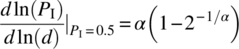Since α is non-negative, (Equations 8.21) and (8.22) always yield slopes less than the respective exponential (Eqs. (8.11) and (8.12)). In other words, the beta-Poisson model is shallower than the exponential model. This is shown in Figure 8.2 in which it is also shown that as α → , the beta-Poisson model approaches the exponential model. Figure 8.2 (top) also shows that all models at sufficiently low doses yield a slope of 1—indicating linearity, on a log–log plot.

Simple Threshold Models

The beta-Poisson dose–response model modifies the exponential model by allowing for a distribution of microorganism–host interaction probabilities. Another way of modifying the exponential model is by stipulating that some minimum number of surviving organisms other than one (i.e., kmin > 1) is required for the infection process to occur. This model has already been given in Equation (8.6). However, the sum of Poisson terms in Equation (8.6) can be replaced by an incomplete gamma function: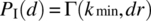This functional form is identical to that for a gamma probability distribution , and therefore, properties of the gamma distribution can be used to make inferences about the threshold models. This “threshold” model was invoked in the context of microbial dose–response assessment (albeit in cell culture assays) as early as Lauffer and Price . Unlike the beta-Poisson, and all mixture models that can be written in the form of Equation (8.14), multihit models with kmin > 1 do not have low-dose linearity (or slopes of 1:1 on log–log plots), and their slope at the median infectious dose is in excess of the simple exponential. On a log–log scale, the slope at low doses is in fact equal to kmin. On an arithmetic scale, the slope of risk is proportional to the kmin power of dose, at low dose. The behavior of these curves is shown in Figure 8.3; the curves are computed such that the median infectious dose is 10 organisms.Figure 8.3 Behavior of threshold models as a function of kmin. All models computed to have a median infectious dose of 10 organisms.

The term “threshold” is used to indicate that, at the level of the interaction between the microbial pathogens and the human host, more than one organism is required to survive to initiate infection. This is the hypothesis of cooperativity, or in the analogy of Meynell and Stocker presented earlier, the multiple bullet scenario. The term “hit” is also used in connection with these models, since they are identical to the “single-target multihit” model used in analysis of radiation dose–response .

In general, threshold models have not been widely used in microbial risk assessment work, since virtually all experimental data show slopes at the median infectious dose equal to or less than the simple exponential. Therefore, the nature of differences most frequently is in the direction shown by Figure 8.2, rather than that shown by Figure 8.3.

Thresholds with Variable Host Sensitivity

The threshold approach can also be used to modify the models that incorporate variable host sensitivity (the beta mixture of r values). We can start with Equation (8.6), for a threshold number kmin. This is now integrated with respect to a beta distribution of r values in the manner of Equation (8.16) to yield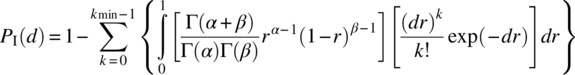Each of the integrals in braces in Equation (8.24) is of the same functional form as in Equation (8.13). Hence, the result can be written as a series of confluent hypergeometric functions as follows:

(8.25)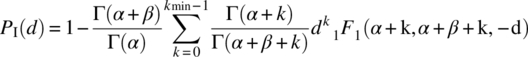On a log–log plot, this equation has a slope of kmin—so it also produces a substantially lower low-dose risk estimate than the nonthreshold (kmin = 1) variants.3

Negative Binomial Dose Distributions

Another possible variant on the general dose–response relationship (Eq. 8.2) is when the actual dose distribution differs from Poisson. This might be due to systematic variability in the medium in which organisms are administered to exposed individuals. We will consider the NB distribution as an alternative to the Poisson; however, any alternative discrete distribution can be used.

Recall that the NB distribution can be obtained as a gamma mixture of Poisson distributions. The dose–response when a mixture of Poisson distributions characterizes the dose distribution can be given by averaging the corresponding Poisson dose–response relationship with the mixture distribution itself. In other words, if PI,Poisson is the dose–response relationship corresponding to the simple Poisson dose-to-dose distribution of microorganisms, then the dose–response relationship assuming a mixture of Poisson distributions with mixing distribution density function h(d) is given byWith Constant Host Sensitivity

If we assume an NB, with constant host sensitivity (constant r), then the effect of non-Poisson dose distributions is obtained by mixing Equation (8.7) (simple exponential) with a gamma distribution. For reasons to become apparent, we will reverse the usual parameter designations in the gamma and describe the distribution of mean dose as (and recall that the average dose delivered would be)Using Equation (8.7) as PI,Poisson along with Equation (8.27) in Equation (8.26) produces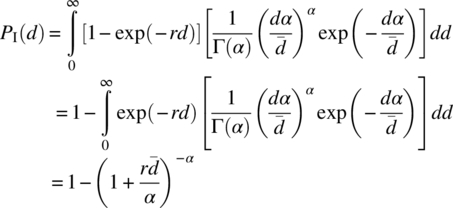Note that the end result of this model is functionally identical to the beta-Poisson model, Equation (8.18). Therefore, if a data set fits Equation (8.18) or 8.28, in the absence of additional information, it is impossible to differentiate between two hypotheses whereby this functional form may be obtained. Furthermore, there is no assurance that additional derivations with alternative assumptions may produce the same model.

With Variable Host Sensitivity

It is also possible to couple the NB dose-to-dose distribution assumption with an assumption of beta-distributed pathogen survival probabilities between doses. Using the approximate beta-Poisson form as PI,Poisson and the gamma mixing distribution as parameterized in the preceding text (except that we use α * for the gamma parameter so that we can retain α as the beta-Poisson exponent), we can obtain the following: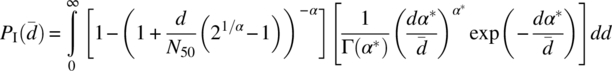Unfortunately, there does not appear to be a closed-form solution to the integral in Equation (8.29). It can, however, be evaluated by numerical integration. Parametric study of this equation, which we do not show, suggests that the incorporation of NB variation in dose-to-dose aliquots has little influence on the shape of the dose–response relationship, either near or at the median infectious dose or at low doses. Therefore, we will not pursue this model further.

Variable Threshold Models

The last class of mechanistic models that will be derived considers the possibility of variable thresholds. That is, suppose the population of persons who are exposed have a distribution with respect to the minimum number of infectious foci that must be initiated prior to a response. In other words, the parameter kmin is characterized by a distribution. Since it is discrete, we must use a discrete distribution. Note that if there are any hosts (persons) for whom kmin equals 0, there will be infections in the absence of any microorganisms; hence, we need to use a discrete distribution with a range of support over the nonzero positive integers.

The starting point for this model is the individual terms from Equation (8.6), giving the fraction of hosts who receive sufficient organisms to initiate k infectious centers (assuming independent identical binomial survival probabilities), [(dr)k /k !]exp(−dr). We consider an infection to occur if the host has a “threshold” of kmin. However, kmin is given by a discrete probability distribution such that P *(kmin) are the fraction of subjects who have a threshold equal to kmin. From this, the total proportion of subjects who receive an actual dose in excess of their “threshold” can be written as

(8.30)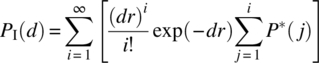There are a number of possible distributions for P *(j). One of the simplest may be the zero-truncated Poisson—which is a Poisson distribution with the zero term remaining. For x = 1, …, ∞,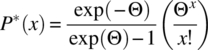When Θ approaches 1, this distribution approaches 1 with 100% weight at x = 1 (i.e., the model in Equation (8.31) approaches the exponential model). Figure 8.4 presents graphs of the resulting dose–response relationship. These graphs are drawn such that the median infectious dose in all cases is 200 organisms. Like the simple “threshold” models, as long as Θ is sufficiently greater than 1, the slope at the median infectious dose is greater than the simple exponential model. However, on a log–log plot, there is a clear change in slope (for higher values of Θ). Low doses result in an effect primarily with those individuals who are most sensitive (having a low kmin). Higher doses must infect the less sensitive individuals (with higher kmin) and therefore result in a higher slope. This is quite different than the pattern in Figure 8.4, in which the slopes at low dose are constant on a log–log plot.Figure 8.4 Dose–response model for constant host–microorganism interaction ratio (r) and truncated Poisson distribution for kmin. All curves fixed at a median infectious dose of 200.

Other Mixture Models

There are clearly many other variants to plausible microbial dose–response models that can be developed. Recalling Equation (8.2), we have a choice of two probability distributions (P1 and P2) as well as of the numerical value or distribution (and associated parameters) used for kmin. The previous discussion outlines some options for these aspects of the model.

In Table 8.1, a taxonomy of dose–response models is presented. A number of these have been presented; however, a number of these models (of greater complexity) can be derived as indicated. In addition, by using a mixing distribution other than the gamma to derive the dose-to-dose variability of delivered microorganisms (e.g., an inverse Gaussian), or by using a distribution other than the beta to describe the variability in r (e.g., Johnson et al.  describe a generalized beta distribution, characterized by three, rather than two, parameters), or by using a distribution other than the truncated Poisson to describe the variability in kmin, a rich spectrum of possibilities emerges. As an indicator of the possible breadth of models, the work of Turner  in developing “hit theory” models for radiation effects can serve as a paradigm.

Biological Arguments for One-Hit Models

As seen, for example, in Figure 8.3 and Figure 8.4, there is a considerable difference between one-hit (whether or not there is Poisson dose-to-dose variability or constancy of r) and threshold models. This difference particularly exists with respect to low-dose extrapolation. Comparing the one-hit versus two-hit simple threshold models (Fig. 8.3), the dose corresponding to a risk of 10−4 differs by over one and one-half orders of magnitude between these two models, despite the fact that the models have an identical median infectious dose. For experiments in which small numbers of subjects have been used, and where the doses are close to the median dose, it becomes hard to differentiate acceptability of fit between these models (note the bottom panel of Fig. 8.3). Hence, it is desirable to obtain some additional mechanistic information supporting or refuting the possibility that one organism, administered to a host, is sufficient to initiate an infection.4 Of course, if data are sufficient to support a model that may be derived from a one-organism hypothesis and to reject a model that can be derived from a threshold process, this is supportive of the idea of a single organism sufficing to initiate infection; however, in view of the fact that individual models may be derived from multiple assumptions, the observation that a model is consistent with a set of data does not serve to prove that a particular set of assumptions used to derive the model are true.

Nonetheless, there has been strong evidence for over 60 years that the single-organism hypothesis produces results consistent with observed infection dose–response. This will be elaborated upon in the case studies in this chapter, and subsequently. Some early historical works on this point are the studies of Lauffer and Price on virus , Goldberg et al.  on respiratory bacterial and viral agents, and Meynell and Stocker  on Salmonella.

From a basic point of view, an individual viable organism contains all of the information necessary to reproduce. In fact, some organisms that may not be recognized as viable, so-called VBNC bacteria, may also initiate a disease process . Perhaps the most persuasive lines of evidence for the single-organism hypothesis, other than pure fits to dose–response curves, have been reviewed by Rubin . A number of prior studies have been conducted in which multiple pathogens at low doses have been used to expose susceptible (animal) hosts. After the infection or disease process has been initiated, the relative prevalence of the individual strains in vivo has been assessed. Under the assumption of independence, one would expect (it is argued) that “the compositions of the samples from all [animals] given [a large dose] should be similar to that of the challenge dose; that samples from [animals] given smaller doses should vary in composition from [animal to animal]; and that most samples from [animals] given 1 or <1 [median lethal dose] should each contain only a single variant” . This is in fact what has been found, and therefore, the single-organism hypothesis is regarded as being supported.

Empirical Models

In contrast to the models developed from mechanistic assumptions, it is also possible to use empirical models that lack the criteria of plausibility as defined earlier. These are typically models that have been used for analysis of chemical toxicity. We shall present three of these; however, there are numerous examples. These models stem from ideas initiated with Gaddum , in which it is presupposed that the population of susceptible hosts (human or animal) has an intrinsic tolerance distribution for an adverse agent. If the population is exposed to the agent at a certain level, then all members of the population who have a tolerance less than or equal to the dosed level will exhibit the adverse effect. Hence, the problem of assessing the dose–response curve is simultaneously that of assessing the tolerance distribution of the susceptible population.

We do not consider these models intrinsically plausible (although as will be seen, certain tolerance distributions may be developed from biologically plausible rationale). Furthermore, there is no assurance that at some point a derivation of certain of the tolerance distribution models will not be found and thereby render it biologically plausible—but no such derivation for the three empirical models is currently known to exist.

A tolerance distribution for microbial dose–response is a probability density function with respect to microbial dose (average number of organisms in a dose), f(d). In principle, any density function with support over the positive line can be a tolerance distribution. Then the dose–response curve for infection is defined by the integral:

(8.32)For convenience, to permit easy evaluation of PI(d), it would be desirable if the integral of the tolerance distribution (i.e., the corresponding cdf) were an analytical integral.

In fact, since any of the dose–response functions derived in the previous sections are differentiable with respect to d and since they each have the properties of a cdf, they can be considered as implicitly specifying a tolerance distribution. For example, since the cdf of the gamma distribution is an incomplete gamma function , the simple threshold model (Eq. 8.23) can also be considered to be a tolerance distribution model with gamma-distributed tolerances.

Table 8.2 gives the dose–response relationships for three empirical dose–response models that have received some attention (although primarily for chemical agents [26-29]). There are also a large number of empirical three- and four-parameter models that have been explored for microbial dose–response assessment . The log-logistic model uses, as a tolerance distribution, the log-logistic distribution. The log-probit model uses the lognormal as a tolerance distribution. The Weibull uses the Weibull distribution.

Figure 8.5 compares the three distributions at low doses. All distributions are computed to have the same median infectious dose (200 organisms) and the same 10% infectious dose (100 organisms). At high doses, all three models are quite similar. At low doses, however, while the log-logit and Weibull models are linear on a log–log scale, the log-probit model has a substantial curvature and gives a much lower-risk estimate.Figure 8.5 Log-probit, log-logistic (logit), and Weibull models. All curves have median infectious dose of 200 and 10% infectious dose of 100 organisms in average dose.

Fitting Available Data

Given a set of data on infectivity, or some other endpoint (such as illness or even mortality), we would like to ask some basic questions with respect to dose–response estimation. These will be answered in order:

1. Assuming a given dose–response model, what are the best parameter estimates using the experimental data at hand?
2. How do we determine which, of a set of plausible models, provides the best fit to the data?
3. Is the best-fitting model itself adequate, or is there still a significant amount of unexplained variance?
4. What is the uncertainty in the parameters estimates of a particular model for the data at hand?
5. Are the results from two or more data sets adequately describable by a common set of dose–response parameters?
6. How can we explain lack of fit?

We will address these issues in turn and in the process will also introduce some real dose–response data (as well as some hypothetical data).

Types of Data Sets

In general, the data set available for dose–response analysis is one of two types. In the first type of data, several sets of subjects (either human or animal) are fed replicates with a known mean dose, and the subsequent response, in terms of infection, illness, or mortality, is determined. In the second type of data set, typically resulting from the investigation of an outbreak study, the average dose administered to individual sets of subjects is estimated, and the attack rate in each group is assessed. Both of these data sets can be described using the same nomenclature. To stress the parallelism to the problem of estimating densities in the dilution assay (studied in Chapter 6), a similar nomenclature to that in Table 6.3 is used. Nomenclature for dose–response studies is given in Table 8.3.

It should be noted that while much data is available using human subjects, in some cases, the only data available is animal data. Principles for extrapolating the results from animal studies to human risk assessment have not yet been thoroughly studied. When animal data are used, it is particularly important to perform validation by comparison with outbreak investigations. This issue of validation will be addressed later in the chapter, and examples of animal-to-human extrapolation are given in the later chapters on case studies.

Best-Fit Estimation

A particular dose–response model is selected for study. This model may be characterized by a function, which predicts the proportion of positive responders given dose and values of one or several dose–response parameters. In a generic sense, we can write the predicted response as πi  = PI(d;Θ) where Θ is the set of dose–response parameters. We will also define the response for each set based strictly on the observations as. If the individual subjects have independent responses, then the overall system can be characterized using a similar likelihood framework as was used for analyzing dilution assays.

We will use the symbol Y, in this context, to denote the value of a −2 log-likelihood ratio—we will now term this the deviance. For the dose–response assay in Table 8.3, we can define this deviance statistic as